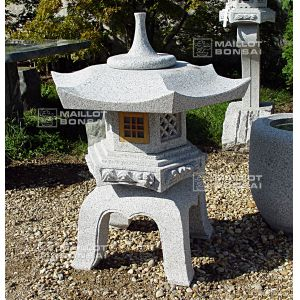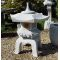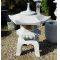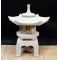##### The Japanese Bonsai specialist
Direct order Contact Help / Services Newsletter# Stone lantern yukimi gata 70 cm wooden window

Yukimi stone lanternref. : 8775

410,00

voluminous and/or heavy product extra shipping of 45,00

Available quantity : 2Order

###### Description

Height 70 cm. Ø 60 cm, weight 80 kilograms, delivered in 5 pieces to be easily assembled at home. A free-standing lantern made of real granite with elegantly curved lines. It features a wooden window, stone latticework and a temple-style pointed roof. An electric cable can be passed through the centre to light a small lamp inside.

#lantern 5.7 #stone 5.5 #wooden 3.6 #window 3.6 #yukimi 2.6 #gata 2.4 #latticework 2.1 #kilograms 1.9 #assembled 1.9 #elegantly 1.9

Formule
(( ROUND((CHAR_LENGTH(b.article_nom)-CHAR_LENGTH(REPLACE(b.article_nom, 'lantern', '')))/LENGTH('lantern')) + ROUND((CHAR_LENGTH(b.article_description)-CHAR_LENGTH(REPLACE(b.article_description, 'lantern', '')))/LENGTH('lantern')) ) * 3.7) + (( ROUND((CHAR_LENGTH(b.article_nom)-CHAR_LENGTH(REPLACE(b.article_nom, 'wooden', '')))/LENGTH('wooden')) + ROUND((CHAR_LENGTH(b.article_description)-CHAR_LENGTH(REPLACE(b.article_description, 'wooden', '')))/LENGTH('wooden')) ) * 3.6) + (( ROUND((CHAR_LENGTH(b.article_nom)-CHAR_LENGTH(REPLACE(b.article_nom, 'window', '')))/LENGTH('window')) + ROUND((CHAR_LENGTH(b.article_description)-CHAR_LENGTH(REPLACE(b.article_description, 'window', '')))/LENGTH('window')) ) * 3.6) + (( ROUND((CHAR_LENGTH(b.article_nom)-CHAR_LENGTH(REPLACE(b.article_nom, 'stone', '')))/LENGTH('stone')) + ROUND((CHAR_LENGTH(b.article_description)-CHAR_LENGTH(REPLACE(b.article_description, 'stone', '')))/LENGTH('stone')) ) * 3.5) + (( ROUND((CHAR_LENGTH(b.article_nom)-CHAR_LENGTH(REPLACE(b.article_nom, 'yukimi', '')))/LENGTH('yukimi')) + ROUND((CHAR_LENGTH(b.article_description)-CHAR_LENGTH(REPLACE(b.article_description, 'yukimi', '')))/LENGTH('yukimi')) ) * 2.6) + (( ROUND((CHAR_LENGTH(b.article_nom)-CHAR_LENGTH(REPLACE(b.article_nom, 'gata', '')))/LENGTH('gata')) + ROUND((CHAR_LENGTH(b.article_description)-CHAR_LENGTH(REPLACE(b.article_description, 'gata', '')))/LENGTH('gata')) ) * 2.4) + (( ROUND((CHAR_LENGTH(b.article_nom)-CHAR_LENGTH(REPLACE(b.article_nom, 'latticework', '')))/LENGTH('latticework')) + ROUND((CHAR_LENGTH(b.article_description)-CHAR_LENGTH(REPLACE(b.article_description, 'latticework', '')))/LENGTH('latticework')) ) * 2.1) + (( ROUND((CHAR_LENGTH(b.article_nom)-CHAR_LENGTH(REPLACE(b.article_nom, 'kilograms', '')))/LENGTH('kilograms')) + ROUND((CHAR_LENGTH(b.article_description)-CHAR_LENGTH(REPLACE(b.article_description, 'kilograms', '')))/LENGTH('kilograms')) ) * 1.9) + (( ROUND((CHAR_LENGTH(b.article_nom)-CHAR_LENGTH(REPLACE(b.article_nom, 'assembled', '')))/LENGTH('assembled')) + ROUND((CHAR_LENGTH(b.article_description)-CHAR_LENGTH(REPLACE(b.article_description, 'assembled', '')))/LENGTH('assembled')) ) * 1.9) + (( ROUND((CHAR_LENGTH(b.article_nom)-CHAR_LENGTH(REPLACE(b.article_nom, 'elegantly', '')))/LENGTH('elegantly')) + ROUND((CHAR_LENGTH(b.article_description)-CHAR_LENGTH(REPLACE(b.article_description, 'elegantly', '')))/LENGTH('elegantly')) ) * 1.9)

## Secure payment## Delivery

Our logistic partners :